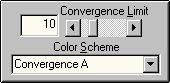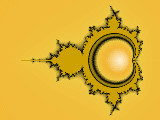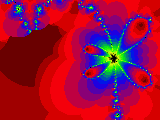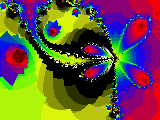PHOTOSWIZZLE Fractals Help - Color Scheme## Color Scheme

The Color Scheme is the fundamental way that colors are chosen for each pixel to render a graphical image after the fractal equations are calculated. Two color selection methods are used by Fractals and these are called Convergence and Divergence. Without getting too much into the technical details of how this is done, here's a brief explanation of what Convergence and Divergence mean.

The fractal calculations are done as a series of iterations on the X-Y coordinates of each pixel in the image. The result of each calculation is a new set of X-Y coordinates somewhere in magical X-Y space. The new coordinates may or may not be within the bounds of the image.

With the Convergence method, the distance between the old coordinates and the new coordinates is calculated at every iteration. If the distance is less than the selected Convergence Limit, the pixel has converged and no more calculations are done on it. In other words the pixel has settled down to a very small area and isn't going to move any more so we don't want to do any more calculations. The number of calculations that were done to get to that point are counted and kept track of.

With the Divergence method, the distance from the center of the image to the new coordinates is calculated at every iteration. If the distance is greater than a fixed number based on the image size, the pixel has diverged and no more calculations are done on it. In other words the pixel has left the playing field and isn't coming back, so we don't want to do any more calculations. Again as with Convergence the number of calculations that were done to get to that point are counted and kept track of.

Now, keeping in mind how these numbers are calculated, read about the Color Palette to see how they are used to assign a color to each pixel.

A few other things to know about the Color Schemes...there are two Convergence choices for the Color Scheme, Convergence A and Convergence B. Convergence B is an interesting variation of the typical convergence scheme. If you experiment with it you will find that it tends to "fracture" parts of the image when compared to Convergence A. There's not much more to say about it without going into mathematical details, so here's an example of both color schemes used with GoldenBrot from the Fractal Gallery:Color Scheme = Convergence AColor Scheme = Convergence B

Also, the Convergence Limit is only used with the Convergence schemes and not for Divergence. Basically the Convergence Limit value is used in an inverse power of 2 relationship (i.e. limit = 1/2n). What does that mean? It means that the larger the control value is, the smaller the distance between coordinates on successive iterations must be to satisfy the convergence criteria. It's not really important to understand this, just that the tendendency is for the image detail to get more complex as the Convergence Limit number gets larger. Here's an example of Flutter By from the Fractal Gallery with two different convergence limits:Convergence Limit = 4Convergence Limit = 6

```
```